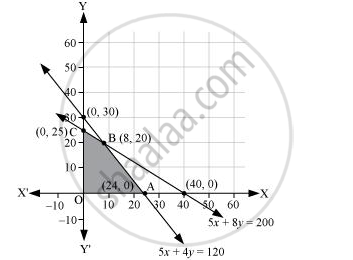Share

# A Company Manufactures Two Types of Novelty Souvenirs Made of Plywood. Souvenirs of Type a Require 5 Minutes Each for Cutting and 10 Minutes Each for Assembling the Profit is Rs 5 Each for Type a and Rs 6 Each for Type B Souvenirs. How Many Souvenirs of Each Type Should the Company Manufacture in Order to Maximize the Profit? - CBSE (Science) Class 12 - Mathematics

ConceptDifferent Types of Linear Programming Problems

#### Question

A company manufactures two types of novelty souvenirs made of plywood. Souvenirs of type A require 5 minutes each for cutting and 10 minutes each for assembling. Souvenirs of type B require 8 minutes each for cutting and 8 minutes each for assembling. There are 3 hours 20 minutes available for cutting and 4 hours of assembling. The profit is Rs 5 each for type A and Rs 6 each for type B souvenirs. How many souvenirs of each type should the company manufacture in order to maximize the profit?

#### Solution

Let the company manufacture x souvenirs of type A and y souvenirs of type B. Therefore,

x ≥ 0 and y ≥ 0

The given information can be complied in a table as follows

 Type A Type B Availability Cutting (min) 5 8 3 × 60 + 20 =200 Assembling (min) 10 8 4 × 60 = 240

The profit on type A souvenirs is Rs 5 and on type B souvenirs is Rs 6. Therefore, the constraints are

5x+8y<=200

10x+8y<=240 i.e.,5x+4y<=120

Total profit, Z = 5x + 6y

The mathematical formulation of the given problem is

Maximize Z = 5x + 6y … (1)

subject to the constraints,

5x+8y<=200… (2)

5x+4y<=120 … (3)

xy ≥ 0 … (4)

The feasible region determined by the system of constraints is as follows.The corner points are A (24, 0), B (8, 20), and C (0, 25).

The values of Z at these corner points are as follows.

 Corner point Z = 5x + 6y A(24, 0) 120 B(8, 20) 160 → Maximum C(0, 25) 150

The maximum value of Z is 200 at (8, 20).

Thus, 8 souvenirs of type A and 20 souvenirs of type B should be produced each day to get the maximum profit of Rs 160.

Is there an error in this question or solution?

#### Video TutorialsVIEW ALL 

Solution A Company Manufactures Two Types of Novelty Souvenirs Made of Plywood. Souvenirs of Type a Require 5 Minutes Each for Cutting and 10 Minutes Each for Assembling the Profit is Rs 5 Each for Type a and Rs 6 Each for Type B Souvenirs. How Many Souvenirs of Each Type Should the Company Manufacture in Order to Maximize the Profit? Concept: Different Types of Linear Programming Problems.
S